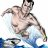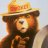# DTR vs ATR Indicator for ThinkorSwim

H

##### New member
@generic thanks for the response and help. what do you mean by "do another?"

G

#### generic

##### Member
@hydroflask Do another as in write another line of code that checks the ATR in a decreasing order. Hope that helps.

D

#### dvorakm

##### New member
2019 Donor
VIP
Found this interesting indicator which provides basic info...however, there is something wrong with the code. The commented lines are the issue. Can anyone figure out how to correct this?

Code:
``````#Study:Info
input ATRLength = 14;
input ShowATR = {default “1”, “0”}; #Average True Range
input AvgVolume = {default “1”, “0”}; #Average volume for the last 14 days
input Volume_ = {default “1”, “0”}; #Volume for today
input ATRPlay = {default “1”, “0”}; #How many ATR’s stock moved today
input VolumePlay = {default “1”, “0”}; #How many of its average volumes stock traded for the last 65 days.
def _ATR_D = TrueRange(high(period = “DAY”), close(period = “DAY”), low(period = “DAY”));
def iATR_D = Round(Average(_ATR_D, ATRLength), 2);
AddLabel (!ShowATR, “ATR(“ + ATRLength + ”) “ + iATR_D, Color.GRAY);
def iAvgVolume = Round(Average (volume(period = “DAY”), 65) / 1000, 0);
AddLabel (!AvgVolume, “AvgVol “ + iAvgVolume + “k”, Color.GRAY);
def iVolume = Round(volume(period = “DAY”) / 1000, 0);
AddLabel (!Volume_, “Vol “ + iVolume + “k”, Color.LIGHT_GREEN);
#def iATRPlay = Round((high(period = “DAY”) — low(period = “DAY”)) / iATR_D, 1);
#AddLabel (!ATRPlay, “ATRPlay “ + iATRPlay + “(“ + Round(high(period = “DAY”) — low(period = “DAY”), 2) + “)”, Color.LIGHT_GREEN);
def iVolumePlay = Round(iVolume / iAvgVolume, 2);
AddLabel (!VolumePlay, “VolPlay “ + iVolumePlay, Color.LIGHT_GREEN);
def Gap = Round((open(period = “DAY”) - close(period = “DAY”)) / close(period = “DAY”) * 100, 2);
AddLabel (yes, “Gap “ + Gap + ”%”, Color.LIGHT_GREEN);
def ATRcur = Round (Average(TrueRange(high, close, low), 5));
AddLabel (yes, “ATR(5) “ + ATRcur, if (ATRcur <= 0.20) then Color.GREEN else Color.RED);``````

This is a description of what the indicator is:

ATR(14) — how much the stock can move per day. Calculated on the average value of 14 days.
AvgVol — average trading volume for a stock over the past 65 days, calculated in thousands.
Vol — how many shares traded today.
ATRPlay — how much today the price has passed relatively from Low to High. It is calculated in dollars and% relative to the average. The screenshot shows that the average price moves \$ 5.5, and today the action has already passed 5.53, the one after ATRPlay is 100%. Without unnecessary symbols to save space. According to my strategy, the potential is no longer there, which means that the share has to be taken in the opposite direction.
VolPlay — What is the volume as a percentage now, the trading volume is relative average. An important parameter for an intraday trader, it is considered as a percentage. 104% on the screen. This means that if you looked at the beginning of the day … This is clearly increased volume. If a stock trades 100% in the first hour, a large seller or buyer is guaranteed to sit there.
Gap — % of gap at the opening is purely informational. I do not analyze in any way, but in some strategies traders rely on it when making a decision.
ATR (5) — And the last parameter is the average price spread for the last 5 bars. If you place a stop, it cannot be less than this value in any way. Otherwise, you will simply be carried away by the noise of the market.#### MerryDay

##### Active member
VIP
@dvorakm The code doesn't present with any syntax errors.#### BenTen

Staff
VIP
@dvorakm I don't see anything wrong with the code. I was able to add it to my chart.#### horserider

##### Well-known member
VIP
Code:
``````#Study:Info
input ATRLength = 14;
input ShowATR = {default “1”, “0”}; #Average True Range
input AvgVolume = {default “1”, “0”}; #Average volume for the last 14 days
input Volume_ = {default “1”, “0”}; #Volume for today
input ATRPlay = {default “1”, “0”}; #How many ATR’s stock moved today
input VolumePlay = {default “1”, “0”}; #How many of its average volumes stock traded for the last 65 days.
def _ATR_D = TrueRange(high(period = “DAY”), close(period = “DAY”), low(period = “DAY”));
def iATR_D = Round(Average(_ATR_D, ATRLength), 2);
AddLabel (!ShowATR, “ATR(“ + ATRLength + ”) “ + iATR_D, Color.GRAY);
def iAvgVolume = Round(Average (volume(period = “DAY”), 65) / 1000, 0);
AddLabel (!AvgVolume, “AvgVol “ + iAvgVolume + “k”, Color.GRAY);
def iVolume = Round(volume(period = “DAY”) / 1000, 0);
AddLabel (!Volume_, “Vol “ + iVolume + “k”, Color.LIGHT_GREEN);
def iATRPlay = Round((high(period = “DAY”) - low(period = “DAY”) / iATR_D), 1);
AddLabel (!ATRPlay, “ATRPlay “ + iATRPlay + “(“ + Round(high(period = “DAY”) - low(period = “DAY”), 2) + “)”, Color.LIGHT_GREEN);
def iVolumePlay = Round(iVolume / iAvgVolume, 2);
AddLabel (!VolumePlay, “VolPlay “ + iVolumePlay, Color.LIGHT_GREEN);
def Gap = Round((open(period = “DAY”) - close(period = “DAY”)) / close(period = “DAY”) * 100, 2);
AddLabel (yes, “Gap “ + Gap + ”%”, Color.LIGHT_GREEN);
def ATRcur = Round (Average(TrueRange(high, close, low), 5));
AddLabel (yes, “ATR(5) “ + ATRcur, if (ATRcur <= 0.20) then Color.GREEN else Color.RED);``````

I got the errors but corrected in above code. Seemed problem was here. Shown in bold.
def iATRPlay = Round((high(period = “DAY”)low(period = “DAY”)) / iATR_D, 1);
AddLabel (!ATRPlay, “ATRPlay “ + iATRPlay + “(“ + Round(high(period = “DAY”)low(period = “DAY”), 2) + “)”, Color.LIGHT_GREEN);

•MerryDay
D

#### dvorakm

##### New member
2019 Donor
VIP
Code:
``````#Study:Info
input ATRLength = 14;
input ShowATR = {default “1”, “0”}; #Average True Range
input AvgVolume = {default “1”, “0”}; #Average volume for the last 14 days
input Volume_ = {default “1”, “0”}; #Volume for today
input ATRPlay = {default “1”, “0”}; #How many ATR’s stock moved today
input VolumePlay = {default “1”, “0”}; #How many of its average volumes stock traded for the last 65 days.
def _ATR_D = TrueRange(high(period = “DAY”), close(period = “DAY”), low(period = “DAY”));
def iATR_D = Round(Average(_ATR_D, ATRLength), 2);
AddLabel (!ShowATR, “ATR(“ + ATRLength + ”) “ + iATR_D, Color.GRAY);
def iAvgVolume = Round(Average (volume(period = “DAY”), 65) / 1000, 0);
AddLabel (!AvgVolume, “AvgVol “ + iAvgVolume + “k”, Color.GRAY);
def iVolume = Round(volume(period = “DAY”) / 1000, 0);
AddLabel (!Volume_, “Vol “ + iVolume + “k”, Color.LIGHT_GREEN);
def iATRPlay = Round((high(period = “DAY”) - low(period = “DAY”) / iATR_D), 1);
AddLabel (!ATRPlay, “ATRPlay “ + iATRPlay + “(“ + Round(high(period = “DAY”) - low(period = “DAY”), 2) + “)”, Color.LIGHT_GREEN);
def iVolumePlay = Round(iVolume / iAvgVolume, 2);
AddLabel (!VolumePlay, “VolPlay “ + iVolumePlay, Color.LIGHT_GREEN);
def Gap = Round((open(period = “DAY”) - close(period = “DAY”)) / close(period = “DAY”) * 100, 2);
AddLabel (yes, “Gap “ + Gap + ”%”, Color.LIGHT_GREEN);
def ATRcur = Round (Average(TrueRange(high, close, low), 5));
AddLabel (yes, “ATR(5) “ + ATRcur, if (ATRcur <= 0.20) then Color.GREEN else Color.RED);``````

I got the errors but corrected in above code. Seemed problem was here. Shown in bold.
def iATRPlay = Round((high(period = “DAY”)low(period = “DAY”)) / iATR_D, 1);
AddLabel (!ATRPlay, “ATRPlay “ + iATRPlay + “(“ + Round(high(period = “DAY”)low(period = “DAY”), 2) + “)”, Color.LIGHT_GREEN);
Thank you! You da man!

L

#### Legitequities

##### New member
Is this measuring the current range abasing the ATR on a daily basis, Periods being days? Can a version be created where it displays the ATR ÷ Current Price of the security to show the ATR % and compare with similar securities?ATR Trailing Stop (5atr period.1.5 factor) crossing 50period moving average with Alarm Questions 0Best ATR ADR Indicator I came up with today (with %) Indicators 0